## Double Ellipsoidal Power Density Distribution

Calculation experience with the ellipsoidal heat source model revealed that the temperature gradient in front of the heat source was not as steep as expected and the gentler gradient at the trailing edge of the molten pool was steeper than experimental measurementse. To overcome this limitation, two ellipsoidal sources were combined as shown in Figure 2-9. The front half of the source is the quadrant of one ellipsoidal source, and the rear half is the quadrant of another ellipsoid. The power density distribution along the £ axis is shown in Figure 2-9.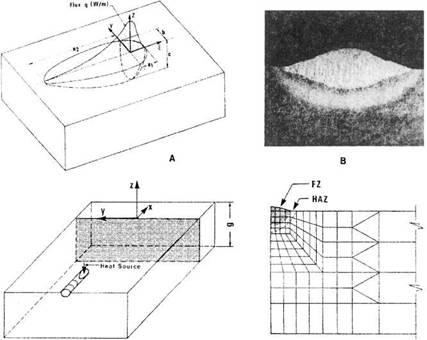Figure 2-9: Double ellipsoid heat source configuration together with the power distribution function along the £, axis (A), Cross-section of an SMAW weld bead

on a thick plate low carbon steel (V=30 volts, 1=265 amps, v=3.8 mm/s, g=38 mm, T0=20.5 °С), Reference plane concept and mesh used for the analysis will be discussed in the following chapters (D).

In this model, the fractions ff and fr of the heat deposited in the front and rear quadrants are needed, where ff +f. = 2. The power density distribution inside the front quadrant becomes:

Similarly, for the rear quadrant of the source the power density distribution inside the ellipsoid becomes:

parameters a, b, с can have different values in the front and rear quadrants since they are independent. Indeed, in welding dissimilar metals, it may be necessary to use four octants, each with independent values of a, b and с

In cases where the fusion zone differs from an ellipsoidal shape, other models should be used for the flux and power density distribution. For example, in welds with a cross-section shaped as shown in Figure 2-10, four ellipsoid quadrants can be superimposed to more accurately model such welds.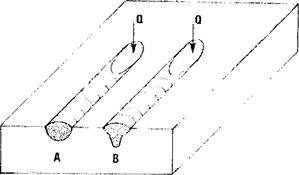Figure 2-10: Cross-sectional weld shape of the fusion zone where a double ellipsoid is used to approximate the heat source (A), compound double ellipsoids must be superimposed to more accurately model such welds.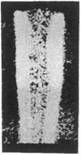Figure 2-11: A conical weld heat source used for analyzing deep penetration electron beam or laser welds; conical source (A), typical electron beam weld (B), cross-sectional kinematic model with reference plane (C) and computed and measured Fusion zone (FZ) and heat affected zone (HAZ) boundaries, (V^70 kV, 1=40 mA, v=4.23 mm/s, g=12.7 mm and T0= 21°C).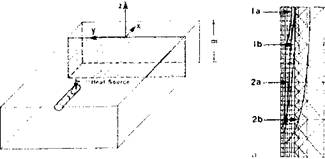о
 The analyst must specify these functions or at least the parameters such as weld current, voltage, speed, arc efficiency and the size and position of the discs, ellipsoids and/or cones. In some cases the weld pool size and shape can be estimated from cross­sectional metallographic data and from weld pool surface ripple markings. If such data are not available, the method for estimating the weld pool dimensions suggested by Christensen  for arc

For deep penetration electron and laser beam welds, a conical distribution of power density which has a Gaussian distribution radially and a linear distribution axially has yielded more accurate results, Figure 2-11.

 POWER DENSITY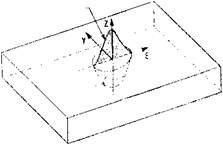welds and by Bibby et al  for deep penetration electron beam or laser welds should be used.

The size and shape of the heat source model is fixed by the ellipsoid parameters defined in Figure 2-10. Good agreement between actual and computed weld pool size is obtained if the size selected is about 10% smaller than the actual weld pool size. If the ellipsoid semi-axes are too long then the peak temperature is too low and the fusion zone too small. The author’s experience is that accurate results are obtained when the computed weld pool dimensions are slightly larger then the ellipsoid dimensions. This is easily achieved in a few iterations. Chakravarti et al  have studied the sensivity of the temperature field to the ellipsoid parameters.

On the one hand these distribution functions can be criticized as “fudge” factors. On the other hand, they do enable accurate temperature fields to be computed. Chosen wisely, varying any parameter changes the computed temperature field. It can be argued that they are needed to model the many complex effects that are quantitatively known, such as electrode angle, arc length, joint design and shielding gas composition .

Комментарии закрыты.